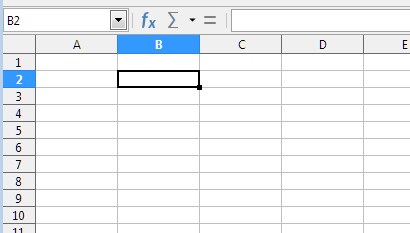Calc function "=HERE()"

Hi

I’m looking to write a function named “=HERE()”, where when I insert =HERE() in cell B3 I get “B3” as result.

Looks easy for formulate, no to easy to get into the API.

Thanks

Do you mean something like this?Use =CELL("address") in B3 => result “\$B\$3”.

Though I didn’t get what you mean with “to get into the API”.

Oh… That easy!
I was looking to get the address from the API such as ThisComponent.Sheet(0).address_where_the_formula_is_and_return_it

To only get the requested location (e.g. B3) without the \$ one can combine the CELL function with the SUBSTITUTE function like this: